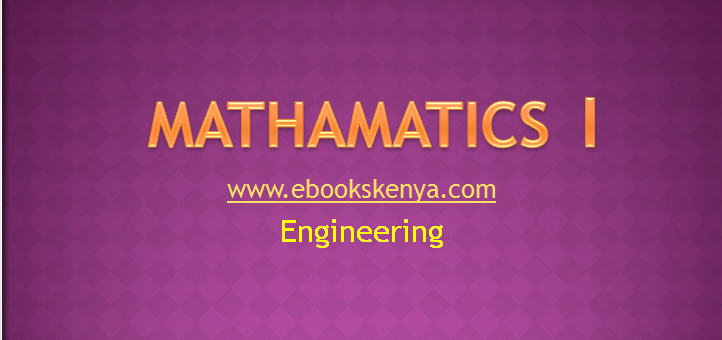# Mathematics I KNEC notesIntroduction

This module unit is designed to equip the trainee with the relevant mathematical knowledge, skills, techniques and attitudes necessary to enhance better understanding of other analytical units of this course, and at the same time provide the trainee with a firm foundation for further training in the trade

### Fractions and Decimals

• Proper fractions and mixed Numbers
• Conversion of mixed and improper
• Fractions and vice versa
• Application of the knowledge of decimals to engineering problems
• Application of fraction to real life situations
• Conversion of fractions into decimals and vice versa
• Recurring decimals
• Compare fractions

### Indices and Logarithms

• Base and index
• Laws of indices
• Indicial equations ‘logarithm
• Laws of logarithm
• Logarithmic equations
• Conversion of bases
• Use of calculator

### Algebra

• Reduction of equations
• Solution of equations reduced to quadratic form
• Solutions of simultaneous linear equations in three unknowns
• Solution of problems involving AP and GP

### Trigonometry

• Half –angle formula
• Factor formula
• Trigonometric functions
• Parametric equations

### Permutations and Combinations

• Definition of permutation
• Definition of combination
• The factorial notation
• Expressions involving permutations and combinations
• Solution of problems involving permutations and combinations

### Binomial Expansion

• Binomial theorem Power series using binomial theorem Roots of numbers using binomial theorem
• Estimation of errors of small changes using binomial theorem

### Coordinate Geometry

• Polar equations
• Cartesian equation
• Graphs of polar equations
• Normals and tangents

### Hyperbolic Functions

• Definition of hyperbolic equations
• Properties of hyperbolic functions
• Evaluation of hyperbolic functions
• Hyperbolic identities
• Osborne’s Rule
• Achx+bshx=C equation

### Inverse Functions

• One to-one relationship in functions
• Inverse functions for one-to-one relationship
• Inverse functions for trigonometric functions
• Graph of inverse functions
• Inverse hyperbolic functions

### Complex numbers

• Definition of complex numbers
• Stating complex numbers in terms of conjugate argument and modulus
• Representation of complex numbers on the Argand diagram
• Arithmetic operation of complex numbers
• Application of Demoivre’s theorem
• Application of complex numbers to engineering
(Visited 4,182 times, 1 visits today)

## 6 thoughts on “Mathematics I KNEC notes”

1.Johnny says:

Very nice course

1.Johnny says:

Nice mathematics

2.permi kim says:

In need of the notes

3.frank ndoch says:

Good

4.Bravin stalon says:

5.Allan kipruto says: